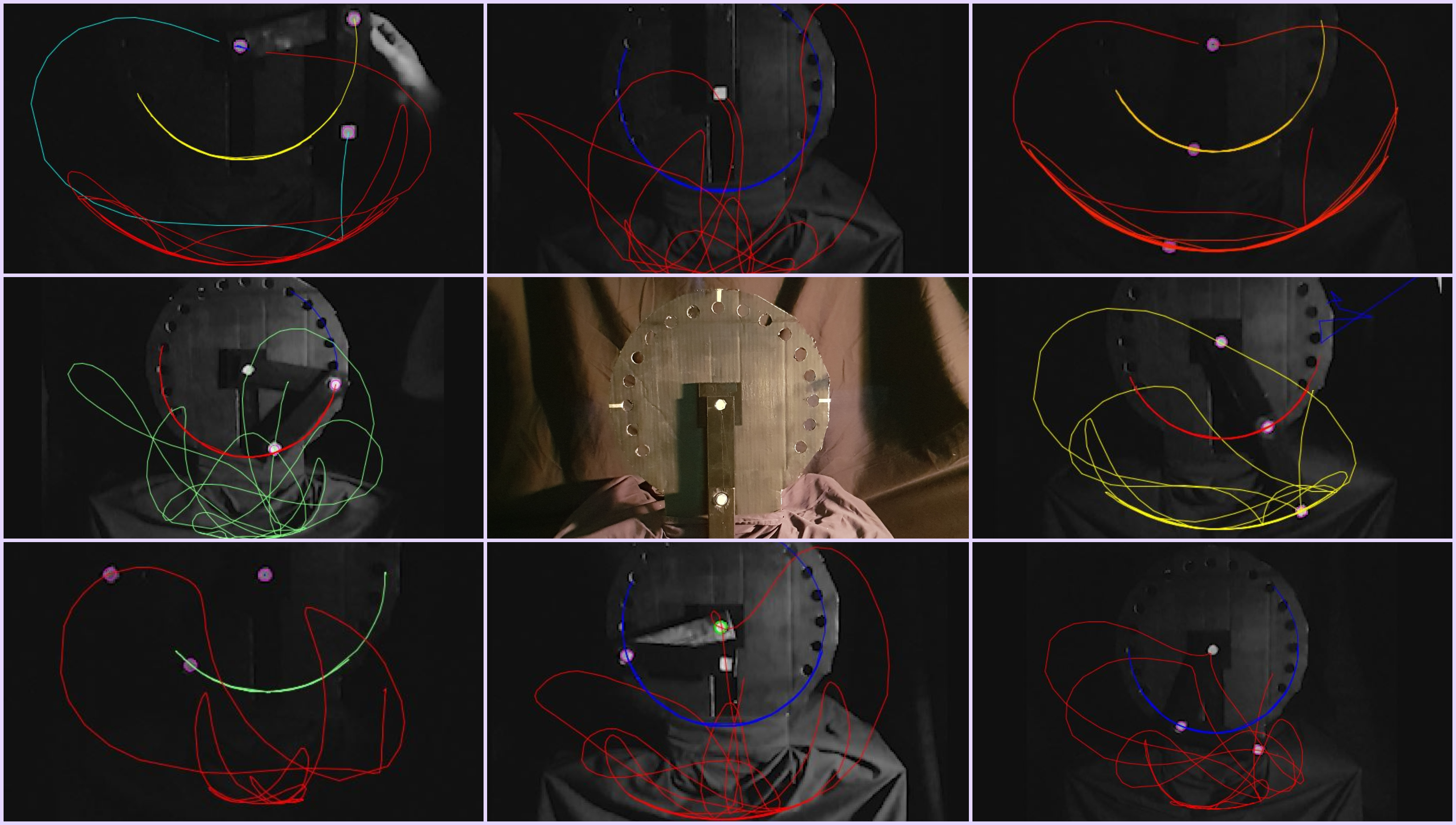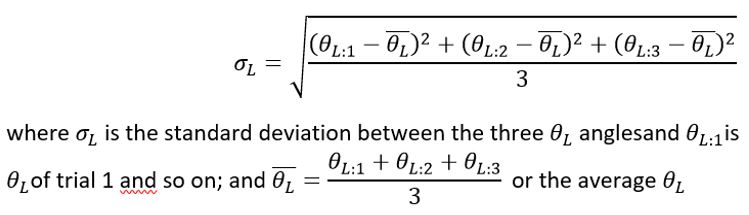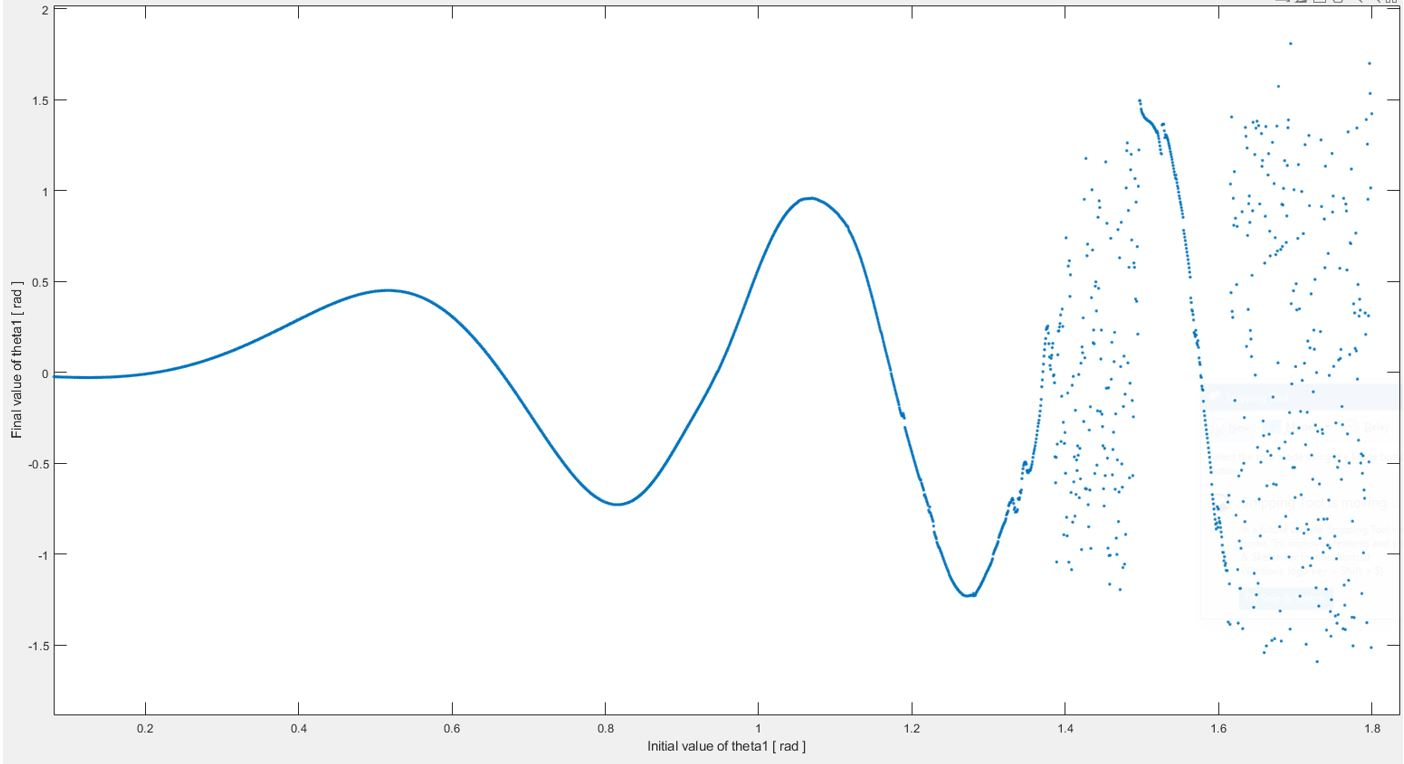# Why Science Can't Tell The Future Part 2

#### October 16, 2021## Processing the data: what does it all mean?

To quantify the difference between the different paths each trial takes I calculated the standard deviation between each value of the lower angle (theta L) for each frame for each set of 3 trials. In nonchaotic motion, when the trajectories of the trials were very similar, the standard deviation between the angles at each frame remained low, whereas in chaotic motion the trajectories diverged and the deviation between the angles was correspondingly high despite the fact each trial the pendulum was released from nearly the same initial position. This is the math I used:Showing how the tracks of different trials released from the same angle diverse by calculating the standard deviation, or difference, between the angles.

Now it gets interesting. If you plot those standard deviations for different initial angles, you get some interesting graphs. Read the axes carefully.

Figure 14 plots the angles whose phase spaces are very similar as was pointed out with the phase spaces already: 90°, 105°, 120°, and 150°. Compared to Figure 15 their deviations are very low. Figure 15 shows this divergence of 135°, 165°, and 180°. An important observation here is the exponential increase of deviation between the tracks, which can be explained that as the tracks become increasingly different, their future trajectories also differ and in turn again the current state of the next frame reflects that and so on. This feedback loop causes exponential divergence of the paths and is another tell-tale sign of chaotic systems.

Another fundamental difference between the deviations of the chaotic vs the non-chaotic sets is that the non-chaotic sets’ trajectories do occasionally reach higher values of standard deviation in what looks like peaks. They then decrease to valleys, and then increase again. This is periodicity. The tracks are not identical frame by frame, but they never exceed a standard deviation of 30, and are therefore very similar not taking time into account, but just slightly offset. The chaotic sets do not show this periodicity except after about frame = 155 when the system loses energy and its motion resembles that of a normal pendulum oscillating around its rest position.

Figure 16 puts all of this together. At first glance there seems to be a divide between order and chaos, where order reigns at 120° and before, and chaos in 135° and after. These lower angles show order because the pendulum does not have enough energy for the two arms to interact independently from one another and trigger exponential divergence. At low energies, complex systems can resemble far simpler systems because their parts do not act independently enough, eliminating the feedback and complexity (when many independent parts interact together in different ways) necessary for chaos.

This energy threshold is why at frame 160 all of the trials, regardless of their initial angle and whether they were chaotic or not, enter into low-energy periodic motion that resembles that of a single pendulum. The two arms swing like one.

One other extremely important observation about Figure 16 is that there is an island of order amidst the chaotic region, at 150°. We know the system has enough energy to act chaotically because it does at 135° – so why is it orderly at 150°? A dive into the underlying theory will aid the investigation of this problem.

### Math is the puppet master: simulating a double pendulum on a computer

There is a way of getting the exact motion of a double pendulum, but it's not as clear cut as a normal pendulum. I'm not too qualified to talk about this so I'll keep it brief.

You can model a double pendulum as a very simple system. The first equations are easy enough, involving just angles, lengths, masses, and angular velocities. But you can't continue with Newtonian equations; you have to use the Euler-Lagrange approach which finds a path of motion which minimizes a systems' 'action,' i.e. the combination of potential and kinetic energy. For complex mechanical systems, the E-L equations are much friendlier to work with. It's something you learn later in physics and engineering studies when you start working with more realistic situations.

Long story short, you eventually get these two differential equations you can plug into a numeric solver (I used Ode45) and visualize using MATLAB.MATLAB simulation of a double pendulum starting at various initial angles. This is chaos theory in one picture.

The figure above plots what the lower angle is at the end of a ten second interval of the system’s motion against the initial value of that lower angle. The masses and lengths were kept at an arbitrary constant value. Up to theta1 = 1.35 radians, the system is orderly because a small change in the initial angle leads to an equally small change in the angle after 10 seconds of motion. But in the chaotic part, a tiny change in the initial angle leads to a totally different path and thereby different final angle.

We see the same features in it as we do in Figure 16, namely the predictable order up to a certain point, the transition to complete chaos at a given value, and a window of stability amongst the chaos (where changes in initial angle lead to proportional changes in final angle, at around 1.5 to 1.6 radians).

Admittedly I got a little lazy after this point. I could've tried to simulate the pendulum I actually built to see how it would behave theoretically, but I had other things to do such as going to the gym, watching Stranger Things season 3, even talking to people again.

As mentioned a double pendulum's chaos arises out of the complexity which is evident in the equations of motion, where all of the parameters depend each other in a variety of ways. The fewer degrees of freedom a system has the less sensitive it becomes, and the more predictable. This is why problems in textbooks never have more than a few. Systems such as the stock market have immeasurable degrees of freedom which all interact and thereby demonstrate chaos. A triple pendulum diverges quicker than a double pendulum.

### Final Words

Examining both experimental data and theoretical causes has revealed some interesting and important concepts.

1. First, in a chaotic system the initial conditions have an enormous influence on its long term behavior. Even a rounding error to the 3rd decimal place will inflate to unimaginable differences, and these differences manifest themselves in an exponential fashion.
2. As Derek Muller from Veritasium put it, “chaos puts a fog on how far you can see either direction – in the past [identifying causes] or in the future [making predictions].” Science uses math but that does not mean that it is math. We may know everything in theory, but in reality we can only know as much as our instruments and experiments tell us. Our maps are not the actual place.
3. Chaos and order interplay with one another, just like in the yin and yang. There are regions of order within total chaos, and the reverse can be true as well. The root cause lies in the mathematics of nonlinearity and complexity and energy, topics that requires textbooks just for themselves. But even those books can’t fully explain this counter intuitive reality.

Chaos is everywhere. The observations extracted from this investigation of math and physics are even evident in history, finance philosophy, art, and human temperament. It is awe-inspiring that all of these seemingly different fields tell the exact same story. The concepts outlined in this paper are actually so fundamental I am surprised physics courses even in high school do not include it in their syllabi, which portray the world to be periodic and orderly. Everything that swings has a period T, no air resistance, and rigid massless strings. If you drop a body there’s a constant friction force and it glides precisely according to the kinematic equations. This is not reality.

We can explain the weather to 3rd graders, yet we still don’t know if tomorrow it’ll rain. Science can't tell the future.

Chaos is the dark side of the Yin and Yang that high school science textbooks never tell you about. This is not to say that simple models that omit arguably the most fundamental concept of dynamic systems (chaos versus order) are not necessary – they show us general relationships and tell us approximate things about nature that we otherwise would not know.

However, by that logic is it not also necessary to tip your hat to the other dark, uncertain side of science? It seems to hurt scientists and teachers to admit they really have no idea exactly where something came from, or what everything will look like down the road. But in a strange way not knowing is also kind of beautiful. Think about it this way: at least now we know all the things we don’t know – and that is the first step of learning. Right?

### Sources

• Thumbnail courtesy of Kagee Jay
• Eck,David. "Explore the Mandelbrot Set." math.hws.edu. http://math.hws.edu/eck/js/mandelbrot/MB.html.
• "Equationsof Motion for the Double Pendulum (2DOF) Using Lagrange's Equations."Video, 20:32. YouTube. Accessed August 27, 2020.https://www.youtube.com/watch?v=tc2ah-KnDXw.
• Gleick,James. Chaos. New Ed ed. N.p.: Vintage, 1997.
• Liu,Cixin. The Three Body Problem. Translated by Ken Liu. Remembrance of Earth'sPast. N.p., 2008.
• MATLAB.2020a ed. Version 4. The MathWorks, Inc., 2020. CD-ROM.
• Nielsen,R., B. Nielsen, and E. Have. The Double Pendulum. March 21, 2013. AccessedAugust 27, 2020.https://pdfs.semanticscholar.org/6f0e/82e1e20e8d7632fcbf4502a7cd7b8eef8be1.pdf.
• Origin8. Northampton, MA: OriginLab Corporation, 2008. CD-ROM.
• "TheScience Behind the Butterfly Effect." Video. YouTube. Posted byVeritasium, December 6, 2019. Accessed August 27, 2020.https://www.youtube.com/watch?v=fDek6cYijxI&t=372s.
• Shampine,Lawrence, and Mark Reichelt. "The MATLAB ODE Suite." In MATLAB, 1-22.Accessed August 27, 2020.https://www.mathworks.com/help/pdf_doc/otherdocs/ode_suite.pdf.
• Shindelin,J., and I. Arganda-Carreras. Figi ImageJ. 2012. ImageJ. Accessed August 27,2020. https://imagej.net/Fiji.
• Strogatz,Steven. Nonlinear Dynamics and Chaos. 2nd ed. N.p.: CRC Press, 2015